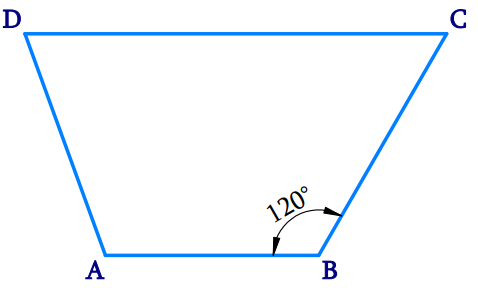# Ex.3.3 Q11 Understanding Quadrilaterals Solution-Ncert Maths Class 8

Go back to  'Ex.3.3'

## Question

Find $${\rm{m}}\,\angle {\rm{C }}$$ in Fig $$3.33$$ if $$AB$$ is parallel to $$DC$$.Video Solution
Ex 3.3 | Question 11

## Text Solution

What is Known?

Given figure is a Quadrilateral with two sides running parallel and one angle is given.

What is Unknown?

Find $${\rm{m }}\angle {\rm{C}}$$

Reasoning:

Trapezium is a quadrilateral with one pair of parallel sides.

Steps:Given figure $$ABCD$$ is a Trapezium, in which $$AB$$ is parallel to $$DC$$.

Here,

\begin{align}\angle {\rm{B}} + \angle {\rm{C}} &= {180^ \circ }\left[ \begin{array}{l}{\text{pair of adjacent }}\\{\text{angles are }}\\{\text{supplementary}}\end{array} \right]\\{\rm{12}}{0^\circ } + \angle {\rm{C}} &= {180^ \circ }\\\angle {\rm{C}} &= {180^ \circ }\\\angle {\rm{C}} &= {180^ \circ } - {120^ \circ }\\\angle {\rm{C }}&={60^ \circ }\\\therefore \,\,m\angle {\rm{C }}&={60^ \circ }\end{align}

Learn from the best math teachers and top your exams

• Live one on one classroom and doubt clearing
• Practice worksheets in and after class for conceptual clarity
• Personalized curriculum to keep up with school